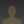# Arduino Based Calculator with Power Boost Moduleakanzler007 wrote 06/29/2020 at 12:15 • 5 min read • Like

DC-DC converters are widely used in portable electronic devices like mobile phones and laptops and are primarily powered with batteries. These applications consist of many sub-circuits which provide different voltage levels to different modules of the system. Today we will see one of the many projects in which a Boost converter comes in handy.

Calculators have become very important in todays time. In schools, colleges and even in many offices calculators are regularly used. They can be used to calulate different things and even in different number systems. Isn’t it exciting to have your own calculator? So, do you want to make your own calculator? Well, today you will get an idea on how to build your own basic calculator.

So let’s get started

• This project consists of DIY based keypad that works perfectly, an Arduino programmed 328p controller, LCD with I2C module, 3.3v to 5v boost circuit and perf-boards.
• Use and solder 8 push buttons which are arranged in such a way that they look like a real Arduino keypad.
• Use a male Berg connectors that can act as a jumper and also it acts as a conductor.
• These connector pins are connected to the Atmega 328p microcontroller with the help of L-shaped male headers. As you can see in the above photos that four pins act as rows pins while the other four pins act as columns.
•    Now, let's discuss the mainboard. The mainboard consists of a micro-controller, a 16Mhz crystal oscillator, two 22pF non-polar capacitors, an I2C connector for LCD and a boost circuit as shown in the circuit below.
• As for the LCD, the I2C module is directly soldered on the LCD PCB, and there is no gap between them.
• The mainboard is powered by the 3.3v LiPo battery, the boost circuit converts the low voltage to the high voltage since there is the potentiometer connected on the boost circuit which varies the voltage at the output.
• So by varying it, the voltage can be changed, this micro-controller only requires about 5V, so the voltage is varied to 5V on the output. This voltage is also supplied on the I2C module this turns on the LCD.

4.      The I2C module on the LCD consists of four pins which are connected in the following ways:5V -------- 5V (output of boost circuit)

·        GND ---- GND (output of the boost circuit)

·        SCL ------ A5 (SCL of Atmega 328p) Arduino

·        SDA ----- A4 (SDA of Atmega 328p) Arduino

Code

Write the code in the Arduino platform and see if the calculator works

#include <LiquidCrystal_I2C.h>

LiquidCrystal_I2C lcd (0x27, 16, 2);

const byte rows = 4;

const byte cols = 4;

char hexKeys [rows][cols] = {

{'1', '2', '3', 'A'},

{'4', '5', '6', 'B'},

{'7', '8', '9', 'C'},

{'*', '0', '#', 'D'}

};

byte rowPins[rows] = {4, 5, 6, 7};

byte colPins[cols] = {0, 1, 2, 3};

long number = 0;

char dynamicKeys;

String string = "";

boolean enterkey_state;

boolean binButton_state;

boolean hexButton_state;

boolean octButton_state;

boolean decButton_state;

void setup()

{

lcd.begin();

lcd.backlight();

lcd.clear();

lcd.setCursor(0, 0);

lcd.print("Initializing");

for (int i = 12; i <= 16; i++)

{

delay(200);

lcd.print("*");

}

delay(3000);

lcd.clear();

lcd.setCursor(0, 0);

lcd.print("Digital");

lcd.setCursor(0, 1);

lcd.print("Calculator");

delay(3000);

lcd.clear();

}

void loop()

{

enterkey_state = false;

binButton_state = false;

hexButton_state = false;

octButton_state = false;

decButton_state = false;

dynamicKeys = kpd.getKey();

if (dynamicKeys)

{

lcd.clear();

lcd.setCursor(0, 0);

lcd.print("|>");

string = string + dynamicKeys;

lcd.print(string);

number = string.toInt();

}

if (dynamicKeys == 'A')

{

octButton_state = true;

lcd.clear();

lcd.setCursor(0, 0);

lcd.setCursor(0, 1);

lcd.print("|>");

lcd.print(number, OCT);

}

else if (dynamicKeys == 'B')

{

binButton_state = true;

lcd.clear();

lcd.setCursor(0, 0);

lcd.print("Binary");

lcd.setCursor(0, 1);

lcd.print("|>");

lcd.print(number, BIN);

}

else if (dynamicKeys == 'C')

{

hexButton_state = true;

lcd.clear();

lcd.setCursor(0, 0);

lcd.setCursor(0, 1);

lcd.print("|>");

lcd.print(number, HEX);

}

else if (dynamicKeys == 'D')

{

decButton_state = true;

lcd.clear();

lcd.setCursor(0, 0);

lcd.print("Decimal");

lcd.setCursor(0, 1);

lcd.print("|>");

lcd.print(number, DEC);

}

else if (dynamicKeys == '#')

{

enterkey_state = true;

lcd.clear();

lcd.print("Entered Number");

lcd.setCursor(0, 1);

lcd.print("|>");

lcd.print(number);

}

else if (dynamicKeys == '*')

{

number = 0;

string = "";

lcd.clear();

lcd.setCursor(0, 0);

lcd.print("Enter Number");

lcd.setCursor(0, 1);

lcd.print("|>");

}

}

Thus we can make many projects with the DC-DC Boost converter module. These modules come in very handy in many projects where you have to increase the voltage needed. Step up boost converter is a DC-DC voltage converter and its main characteristic is the fact that the output voltage is higher than the input voltage. It can very easily and efficiently increase the voltage of a power source to the desired value. Depending on the characteristics, it is possible to increase the input voltage by as much as 10 times!

Get Additional Information on Power Boost Module and make your own project with it.

This guide has been written in reference to the blog posted by create.arduino.cc

## Discussions